## DESIGN AND SIMULATION OF TWO-JOINT PRESSURE-DRIVEN SOFT BIONIC FISH 1)

Jiao Liu, Zhang Baocheng, Zhang Kaisheng,2), Zhao Bo

Department of Mechanical and Electrical Engineering, School of Engineering, Ocean University of China, Qingdao 266100, China

 基金资助: 1)山东省2017年重点研发计划.  2017GHY15015

Received: 2019-04-25   Accepted: 2020-01-24   Online: 2020-05-18Abstract

In order to study and design a new type of bionic robotic fish with high softness and strong environmental adaptability, imitating the shape of shark and the swimming posture of tuna fish, a bionic robotic fish with hydraulic flexible driving structure is designed. In view of the problem that the single-joint hydraulically driven flexible robotic fish has a C-shaped swing posture that does not conform to the swing rule of tuna fish, a two-joint hydraulic flexible drive is used to simulate the S-shaped swing of the fish, and the internal structure of the bionic fish is designed according to the principle of the hydraulic flexible actuator. According to the theoretical wave equation, the swing amplitude of the robotic fish is determined, the magnitude of the pressure load applied inside the flexible actuator is calculated by numerical simulation, and the driving efficiency of the hydraulic flexible actuator is analyzed and calculated. The software of finite element analysis is used to simulate the autonomous swimming process of the robotic fish in the fluid. And the autonomous cruise process of the two-joint robotic fish and the one-joint roboic fish are simulated and compared to obtain the movement postures, swimming velocity and flow field of the two robotic fishes when they autonomously cruised in the fluid. The results show that at the same frequency and tail-fin swing, the average velocity of the two-joint soft roboic fish cruising is 0.29 BL/s, which is higher than the average velocity of the the one-joint roboic fish 0.15 BL/s. And frome the velocity vector diagram, it can be concluded that the S-type swing of the two-joint roboic fish is closer to the real fish swing attitude, and a series of discrete reverse Karman Vortex Streets will be generated during the movement, so the two-joint bionic fish has a higher propulsion efficiency.

Keywords： bionic robot fish ; flexibility ; hydraulic ; swimming performance

Jiao Liu, Zhang Baocheng, Zhang Kaisheng, Zhao Bo. DESIGN AND SIMULATION OF TWO-JOINT PRESSURE-DRIVEN SOFT BIONIC FISH 1). Chinese Journal of Theoretical and Applied Mechanics[J], 2020, 52(3): 817-827 DOI:10.6052/0459-1879-20-001

## 引言

MIT提出的液气压控制的柔性软体机器鱼, 具有单关节柔性鱼身, 能量转换效率高, 鱼身可以产生连续的运动. 但是相比于刚体机器鱼, 其驱动器结构设计、精确建模、精准控制相对困难, 且MIT的单关节柔性鱼身仅能实现C型摆动, 游动效率虽较刚性机器鱼有所提高, 但与自然鱼类相比仍然较低. 本文基于上述分析, 以液压驱动方式设计两关节柔性机器鱼, 采用数值仿真的方法研究了柔性机器鱼的建模和摆动幅值的精准控制, 并基于CEL算法, 建立柔性仿生鱼的自主游动流固耦合有限元模型, 分析比较单关节、两关节柔性机器鱼的游动性能.

## 1 柔性机器鱼结构组成与驱动原理]

### 图1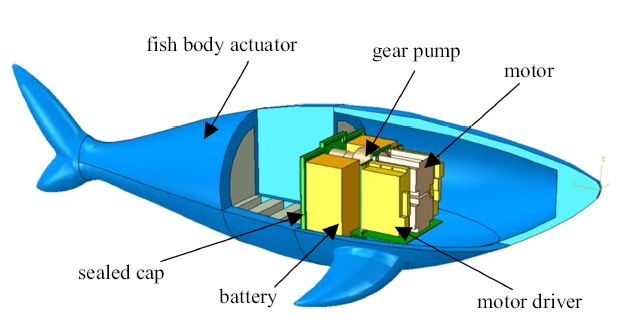Fig.1   The schematic design of hydraulically driven soft robotic fish

### 图2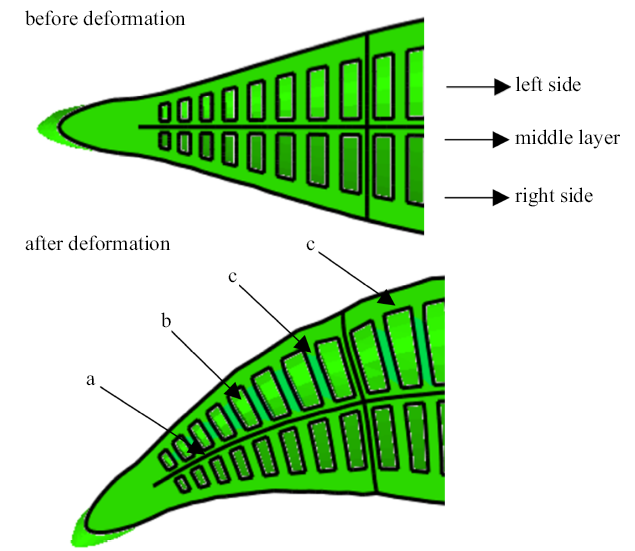Fig.2   The schematic diagram of the fish body actuator before and after deformation

## 2 机器鱼建模

### 2.1 鱼体建模

BCF (body and/or caudal fin)推进模式机器鱼利用身体的变形带动尾鳍摇摆产生推进力向前游动, 游快, 效率高, 得到更多研究员的关注. 其中具有月牙形尾鳍的鲔科模式游动效率最高, 以金枪鱼等硬骨鱼类和鲸鱼、鳖鱼等大型海洋哺乳动物为代表.

### 图3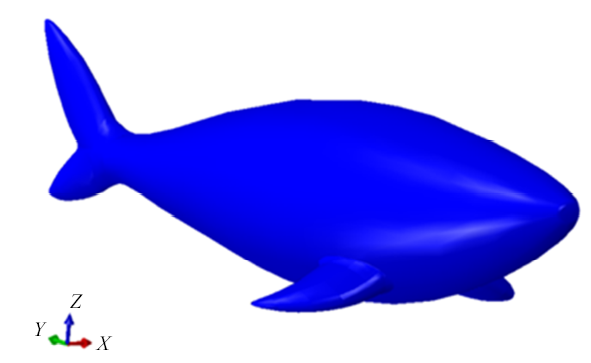Fig.3   Shark-like modeling

### 图4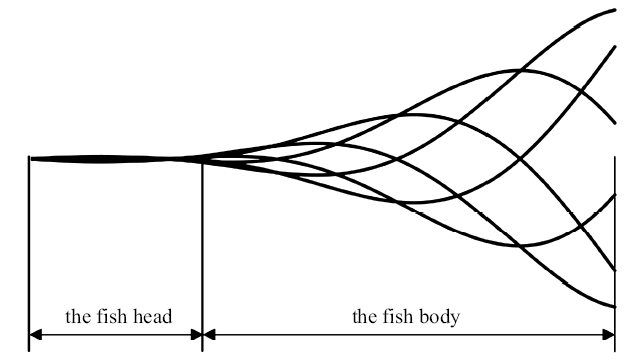Fig.4   The schematic illustration of wavy swimming of tuna fish

### 图5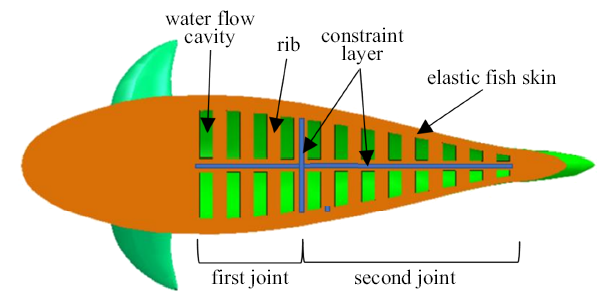Fig.5   The cutaway view of soft fish body actuator

### 图6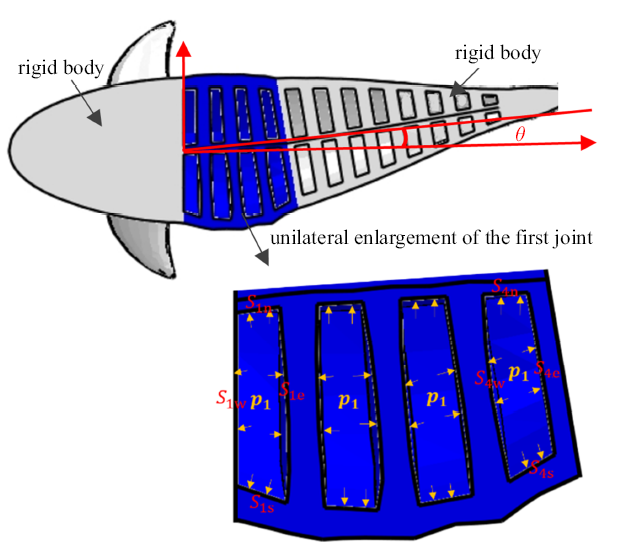Fig.6   Bending diagram of the driver on one side of the single joint

$\theta =0.239 3 p_1^2 +1.973 2 p_1 -0.000 4$

### 图7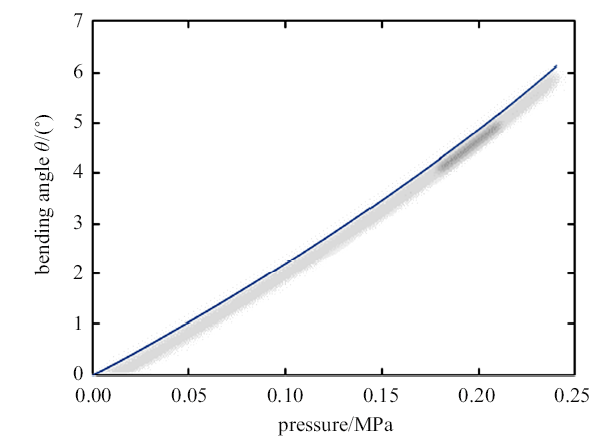Fig.7   Linear relationship between bending angle of soft fish body actuator and internal pressure

### 图8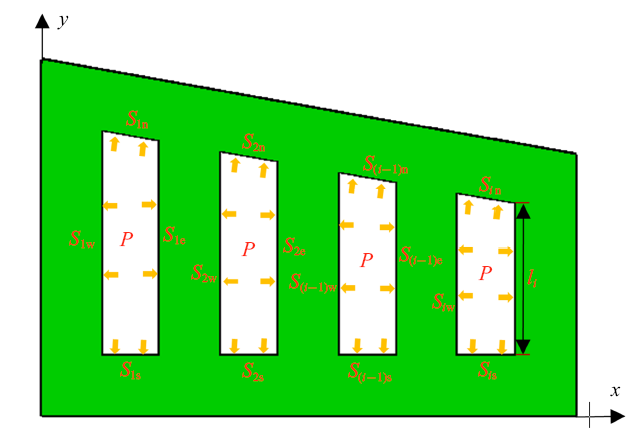Fig.8   Schematic diagram of simplified actuator structure

$M_i =p\cdot S_{id} \cdot l_i$
$A_{id} = A_{ie} -A_{(i+1)w}$

$W_i =p A_{id} l_i\int_{ \theta _{i-1} }^{\theta _i }{{d}\theta }$

$W_{u} =\sum\limits_{i=1}^{n-1} {p A_{id} l_i \int_{ \theta _{i-1} }^{ \theta _i } {{d}\theta } } +p A_{ne} \frac{ l_n }{2}\int_{ \theta _{n-1} }^{ \theta _n } {{d}\theta }$

$\eta =\frac{ W_{u} }{p\Delta V}$

### 图9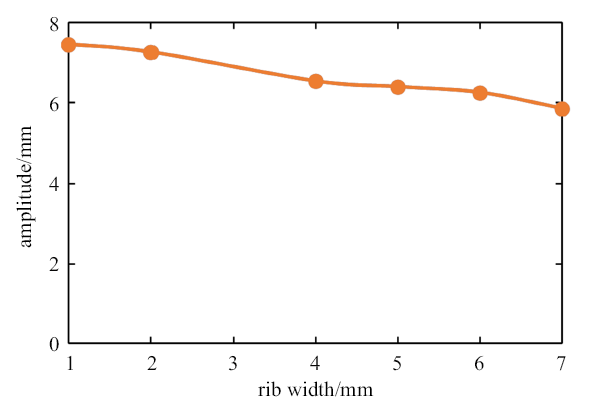Fig.9   The relationship between the width of the rib and the swing amplitude

## 3 仿生机器鱼的体波分析

### 3.1 鲔科鱼的体波描述

$h(x,t)=H(x)\sin (\omega t-kx)$
$H(x)= a_1 + a_2 x+ a_3 x^2$

$h(x,t)=(0.01-0.12x+0.4 x^2 )\sin (4\pi t-11.4x)$

### 图10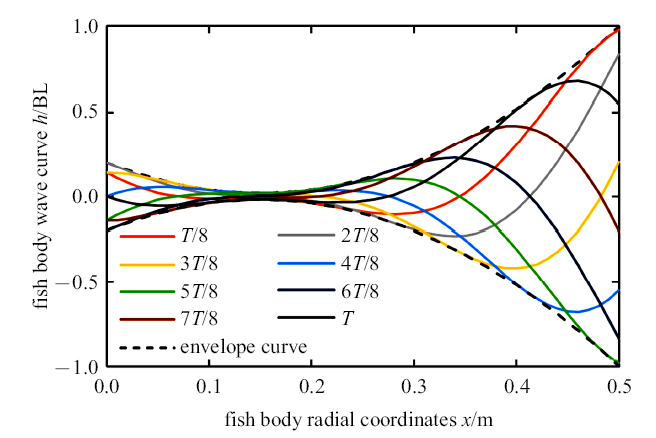Fig.10   The body wave curve in unit swing period

### 图11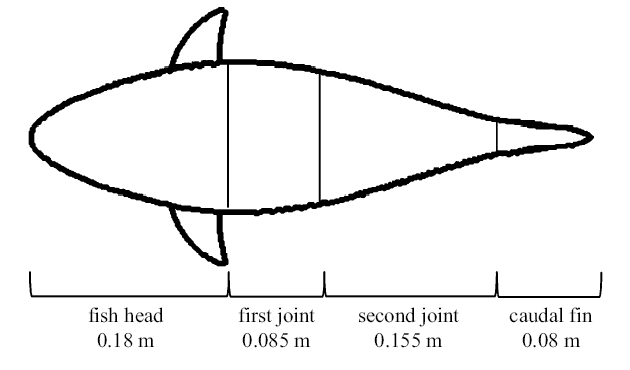Fig.11   The diagram of fish body joint length

$h_1 (t)=0.006\sin (4\pi t-3.021)$

$h_2 (t)=0.03\sin (4\pi t-4.788)$

## 4 动力学仿真

### 图12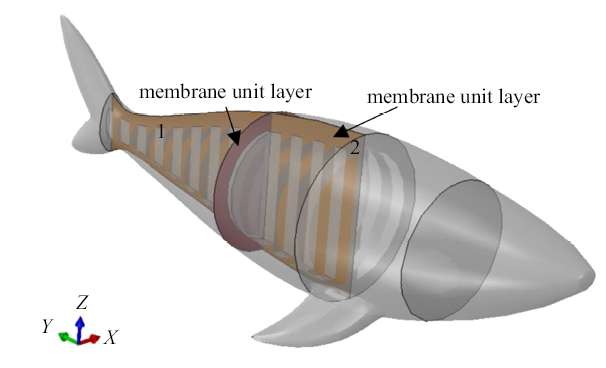Fig.12   The schematic diagram of membrane unit layer

## 5 游动性能分析

### 图13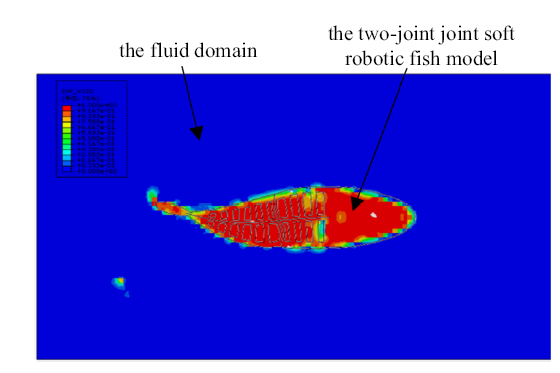Fig.13   The fluid-structure interaction simulation results of two-joint robot fish

### 图14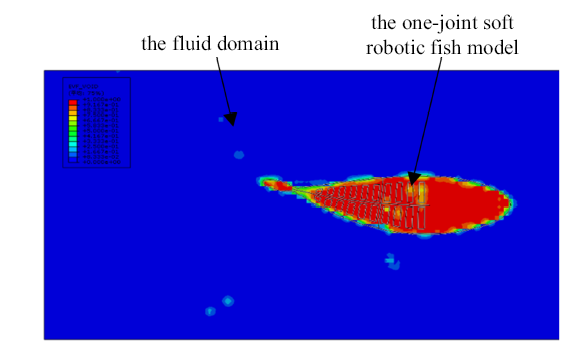Fig.14   The fluid-structure interaction simulation results of one-joint robot fish

### 图15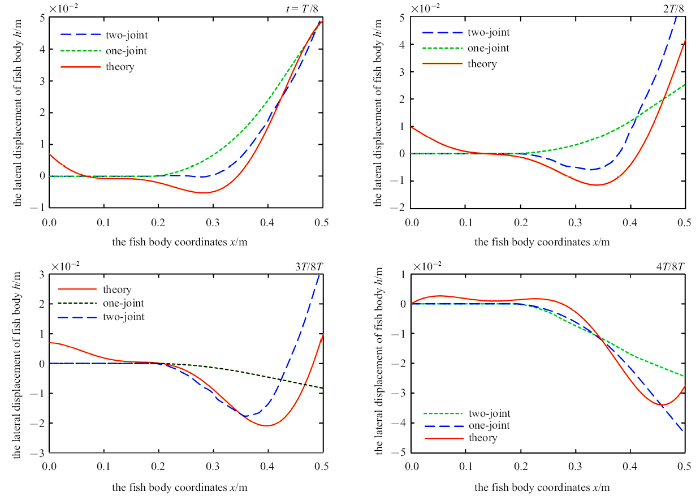Fig.15   Comparison of swing curves in half cycle

### 图16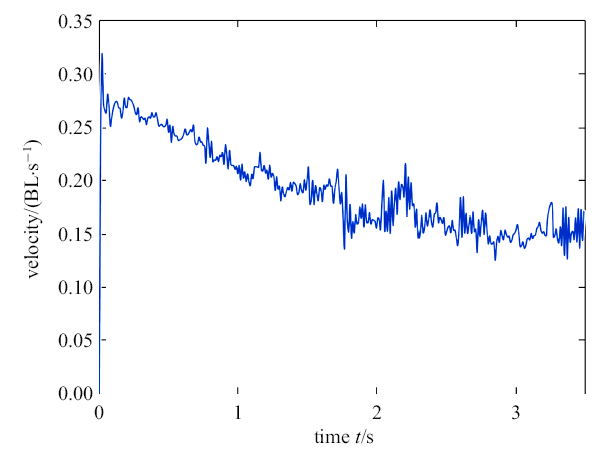Fig.16   The speed-time curve of one-joint soft robot fish during stable swimming

### 图17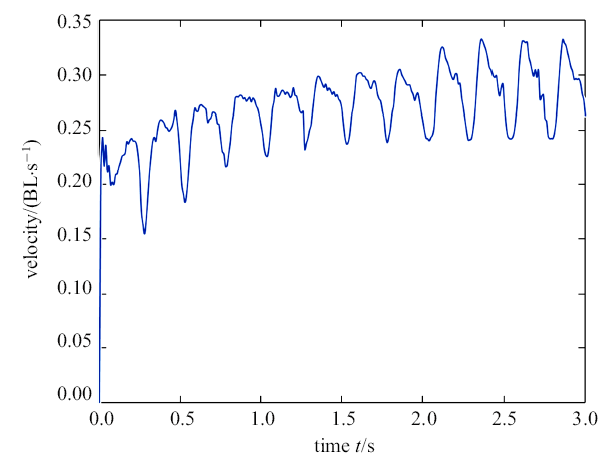Fig.17   The velocity-time curve of two-joint soft robotic fish during stable swimming

Triantafyllou研究发现满足斯特罗哈尔数 (Strouhal) 在0.25 $\sim$ 0.35时, 鱼类在运动过程中会产生一系列离散的反向卡门(Karman)涡街, 其诱导的与鱼体运动方向相反的纵向射流对鱼体运动起推进作用. 也有研究发现采用BCF模式运动加新月形尾鳍推进的鱼类推进效率高的主要因素之一是鱼体游动的$S_t$在0.25$\sim$0.35之间, 可获得最优的推进力和效率. 因此, 本文用无量纲$S_t$比较单关节、两关节机器鱼的推进效率, 其定义式

$S_t =\frac{f\cdot A_f }{U}$

### 图18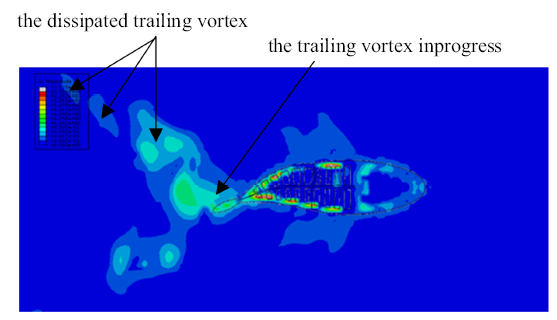Fig.18   The flow field diagram of autonomous swimming of two-joint soft robotic fish

## 6 结论

/

 〈〉